# R S Aggarwal Solutions for Class 11 Maths Chapter 16- Conditional Identities Involving the Angles of a Triangle

Maths is one subject that requires a lot of practice. R S Aggarwal Solutions for Class 11 Maths Chapter 16- Conditional Identities Involving the Angles of a Triangle provides stepwise answers to all the questions from the chapters present in the book. Students should go through the R S Aggarwal Solutions for Class 11 Maths exercises after completing each chapter. These solutions are provided by subject experts at BYJU’S and are accurate.

R S Aggarwal Solutions are very helpful from the examination perspective. Students can refer to the solutions for a better understanding of the topic. The students appearing for the board examinations will find it helpful in scoring well.

### Download PDF of R S Aggarwal Solutions for Class 11 Maths Chapter 16- Conditional Identities Involving the Angles of a Triangle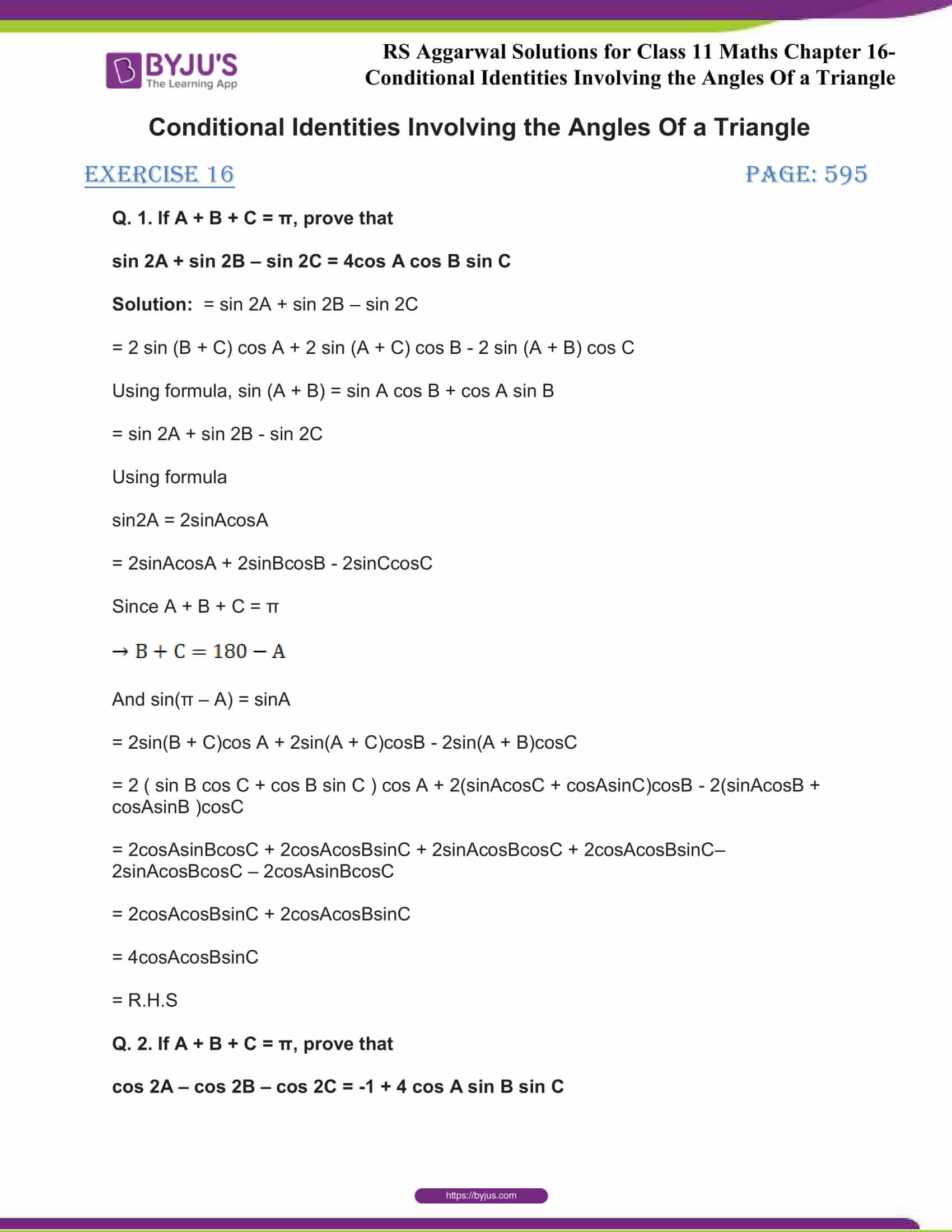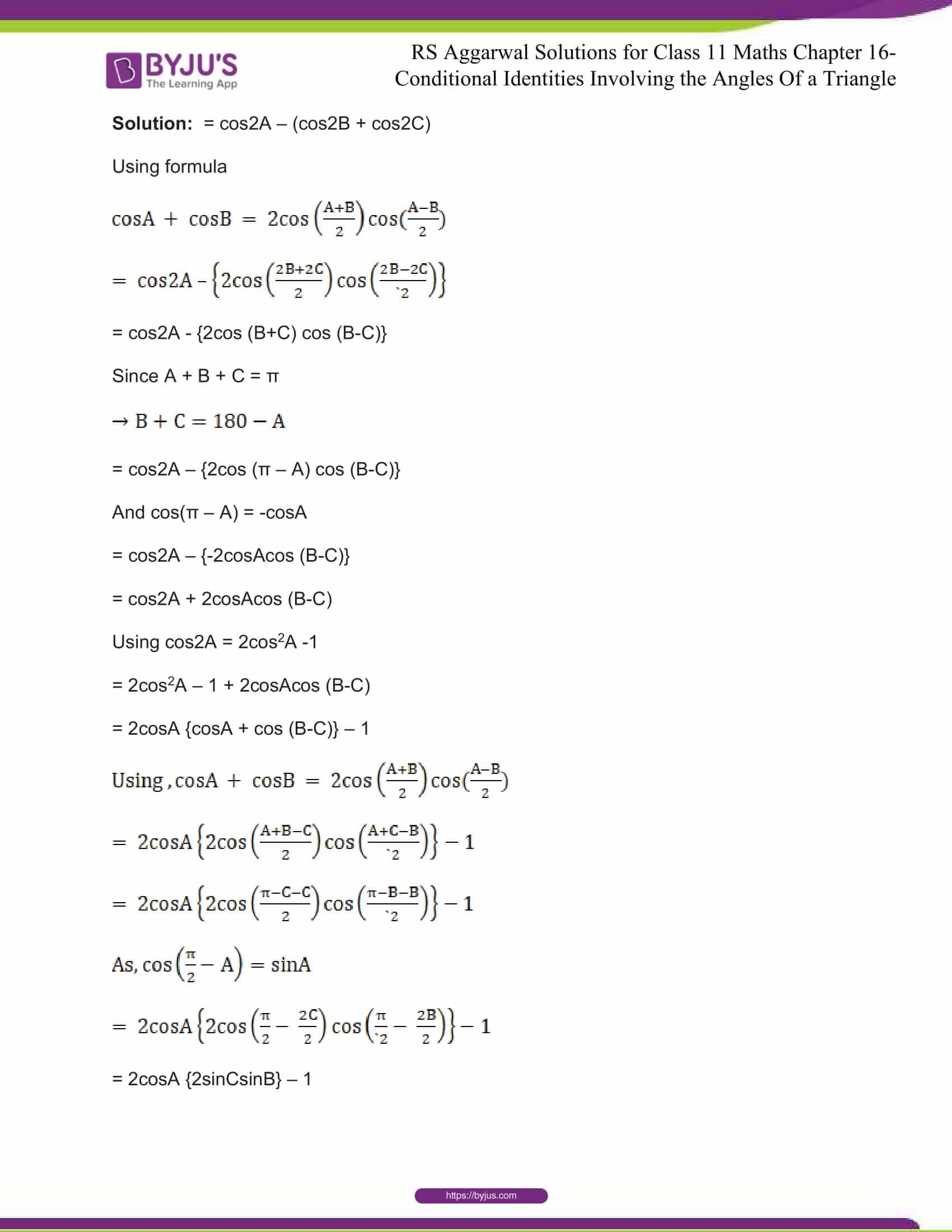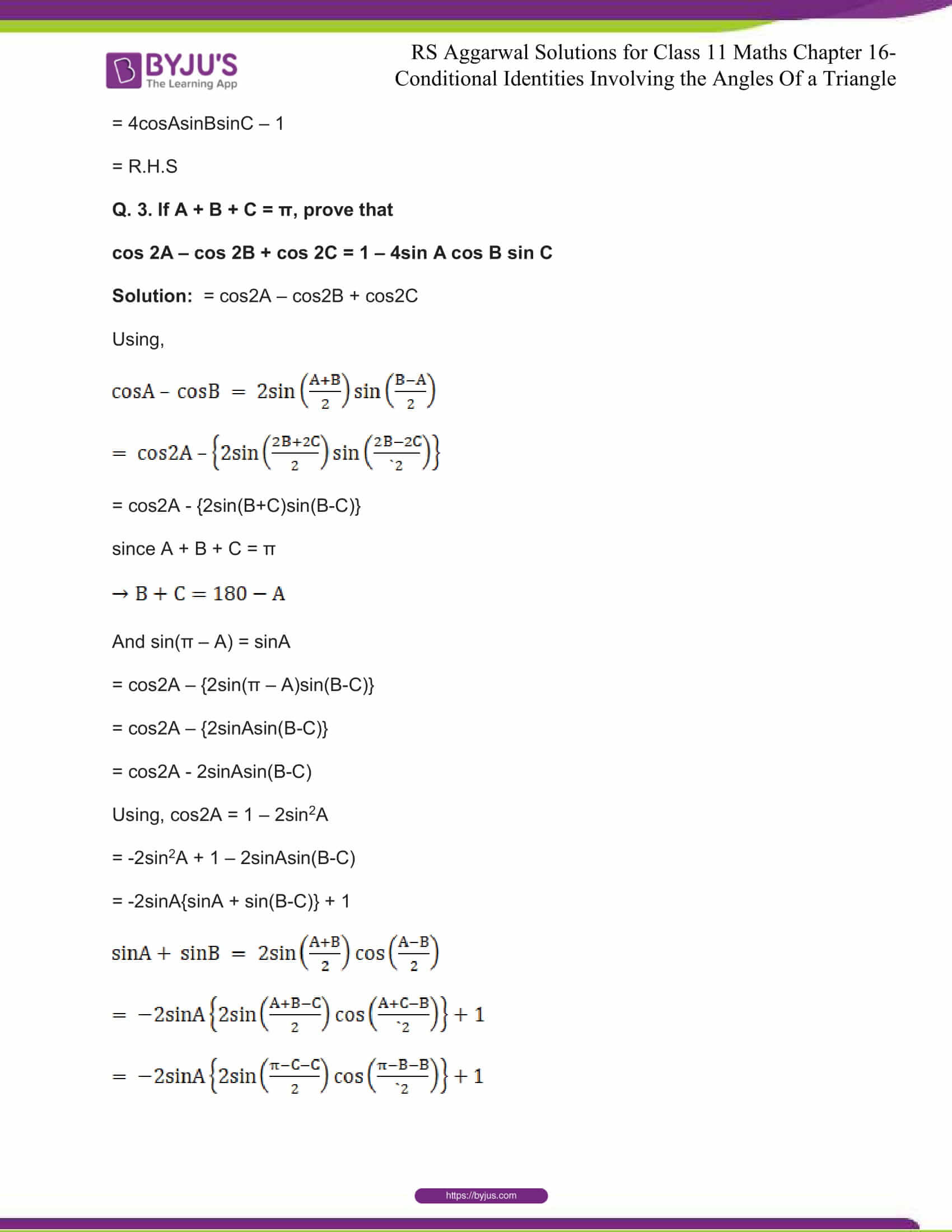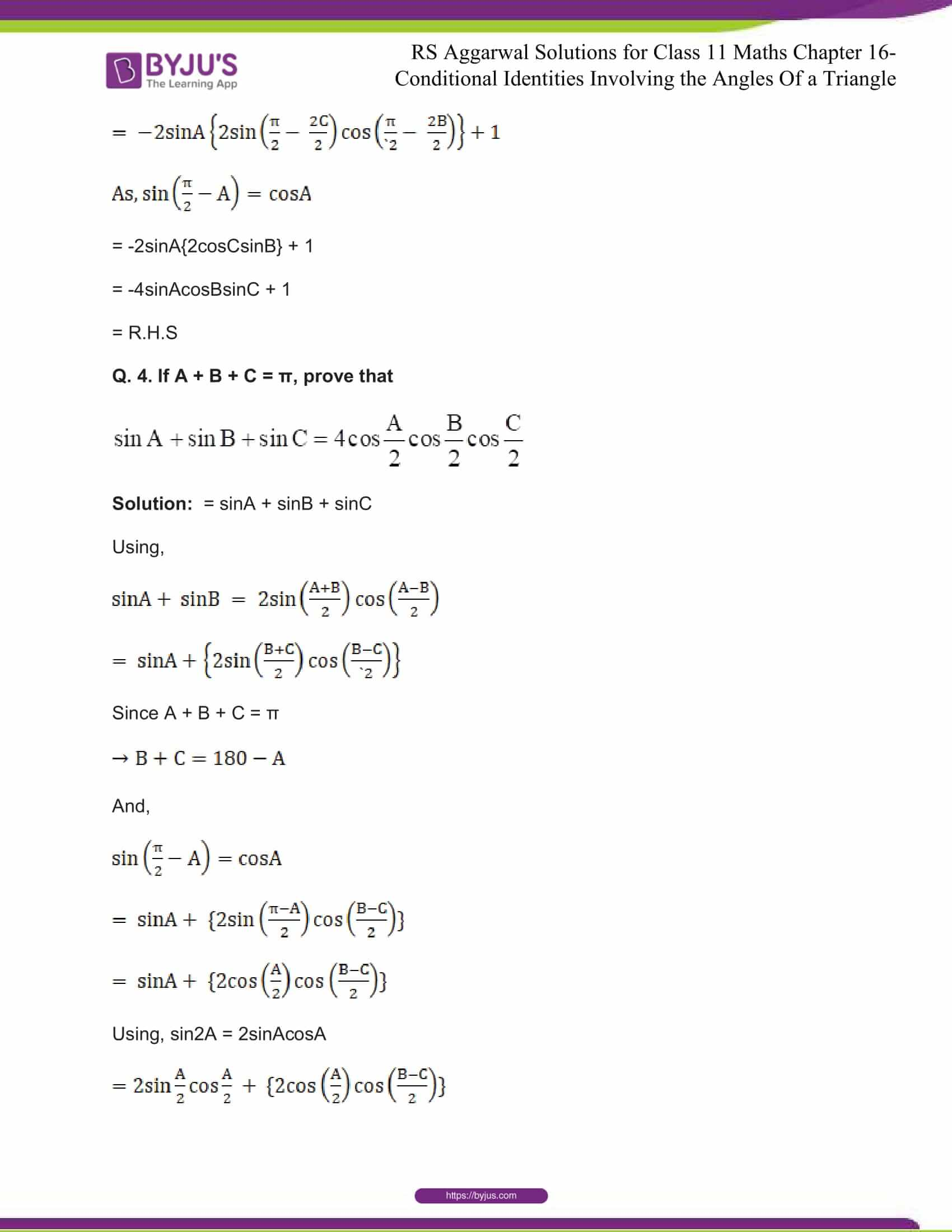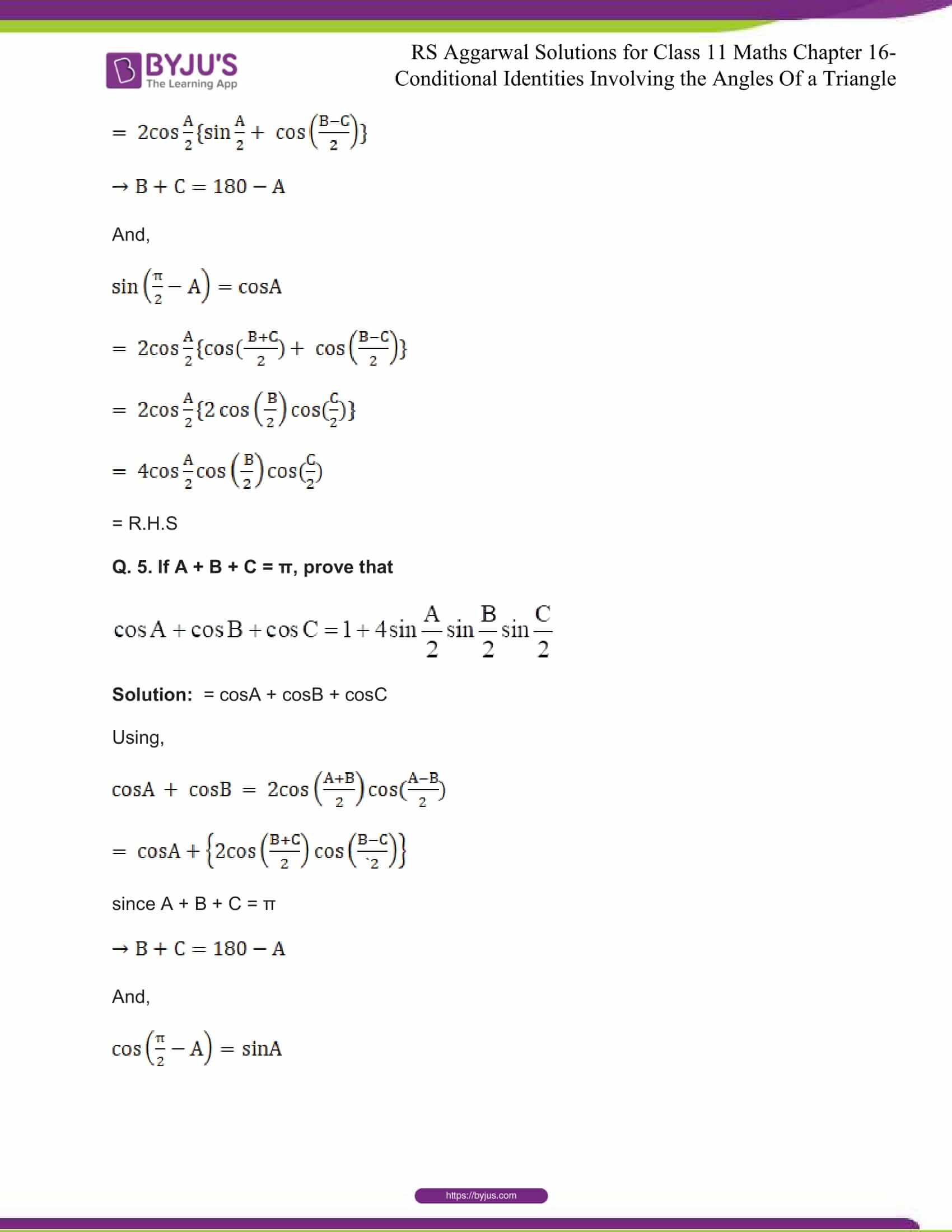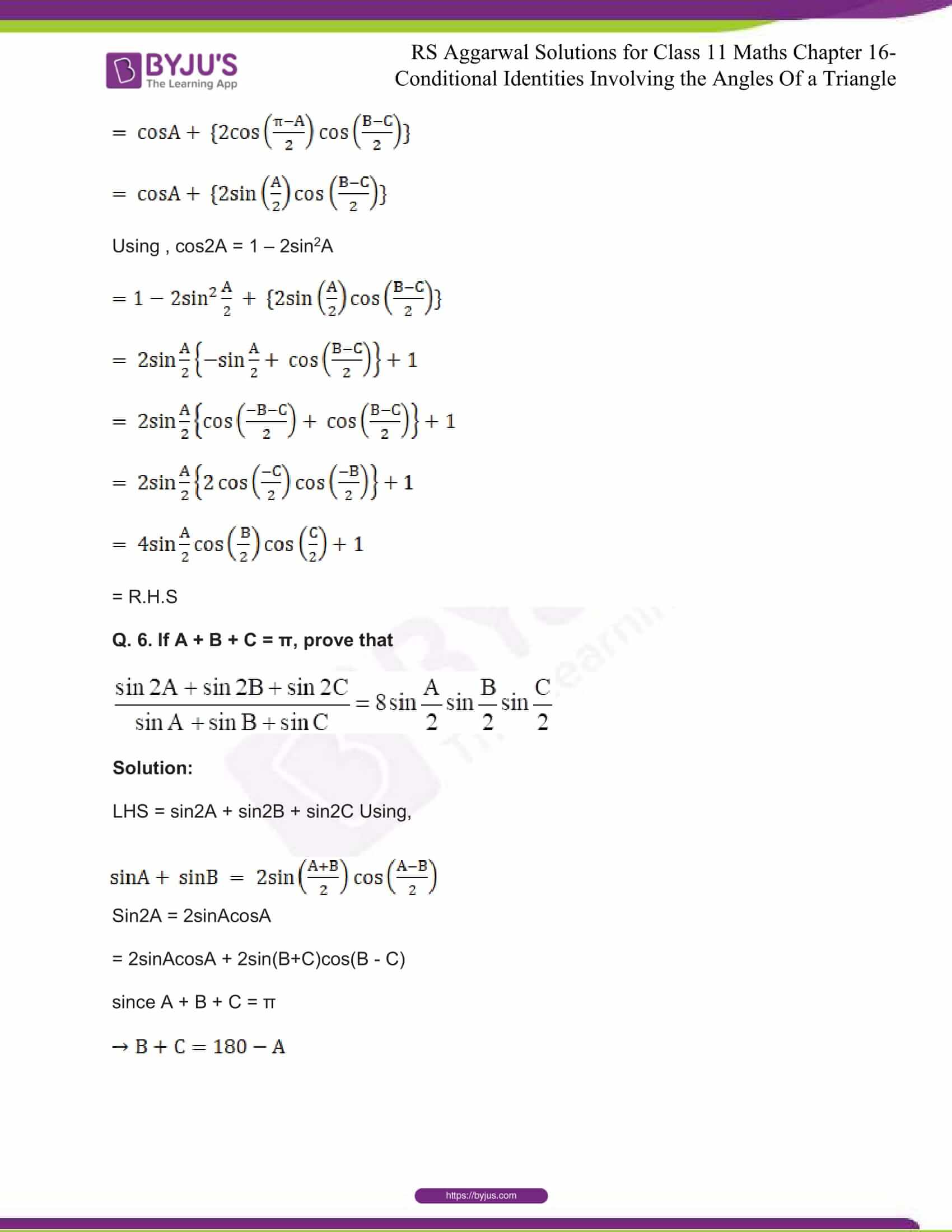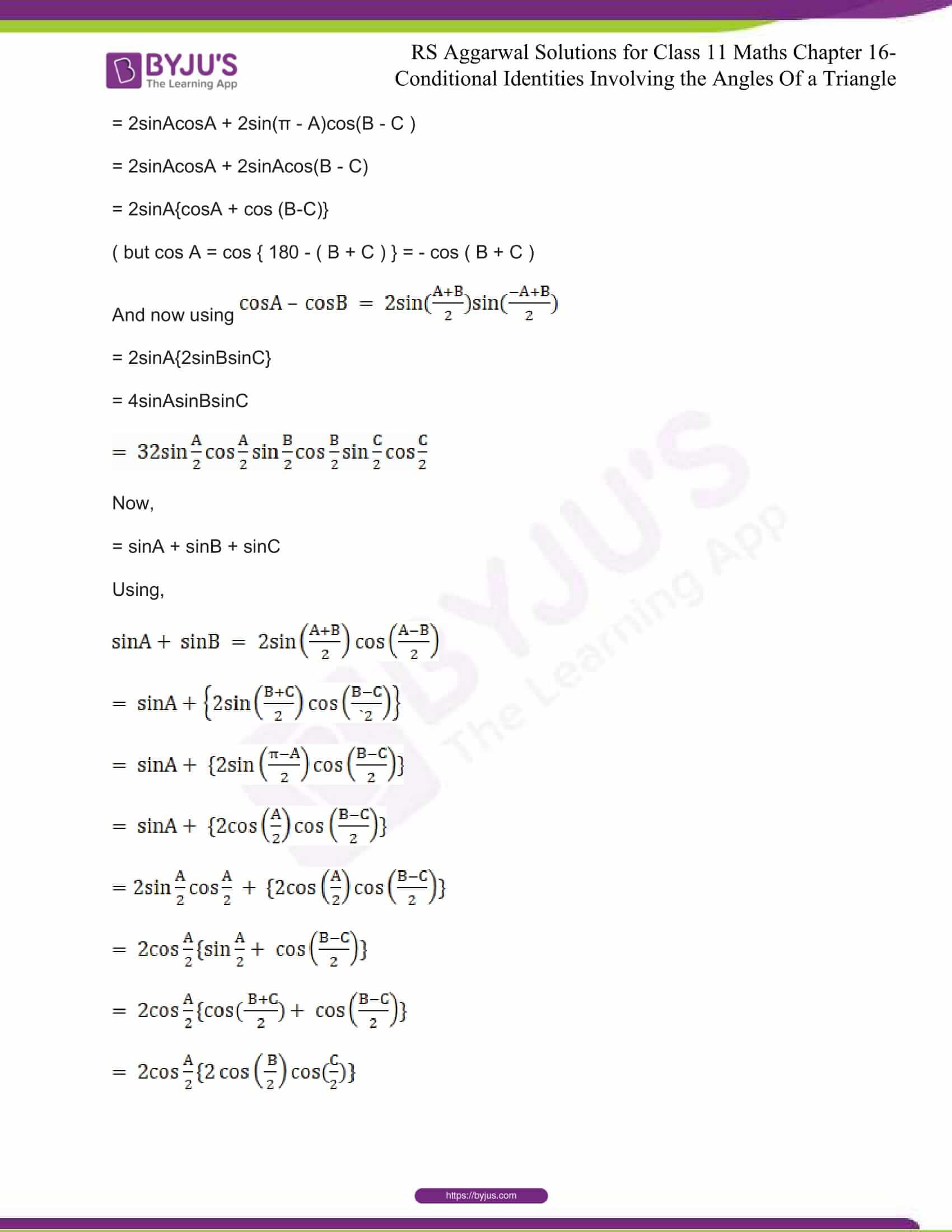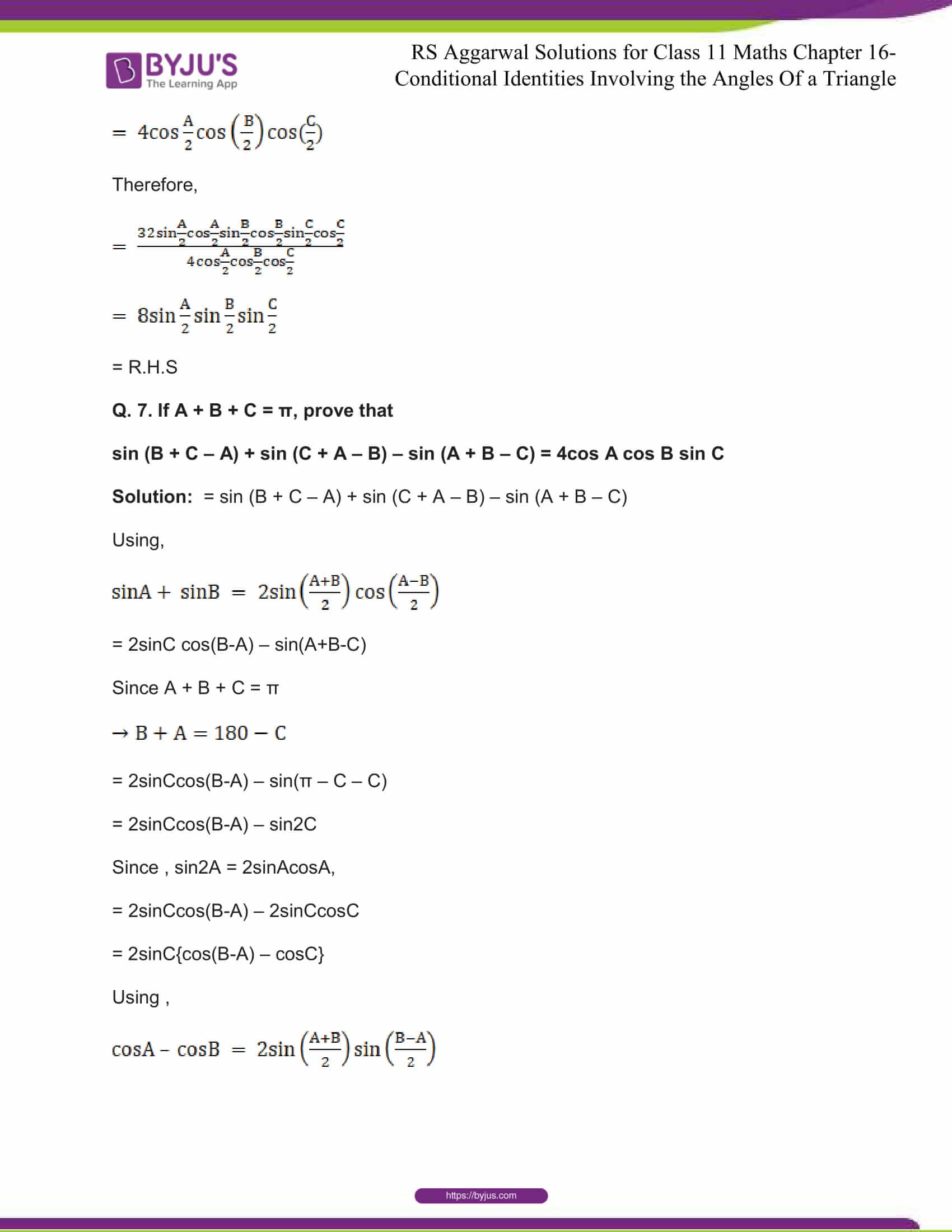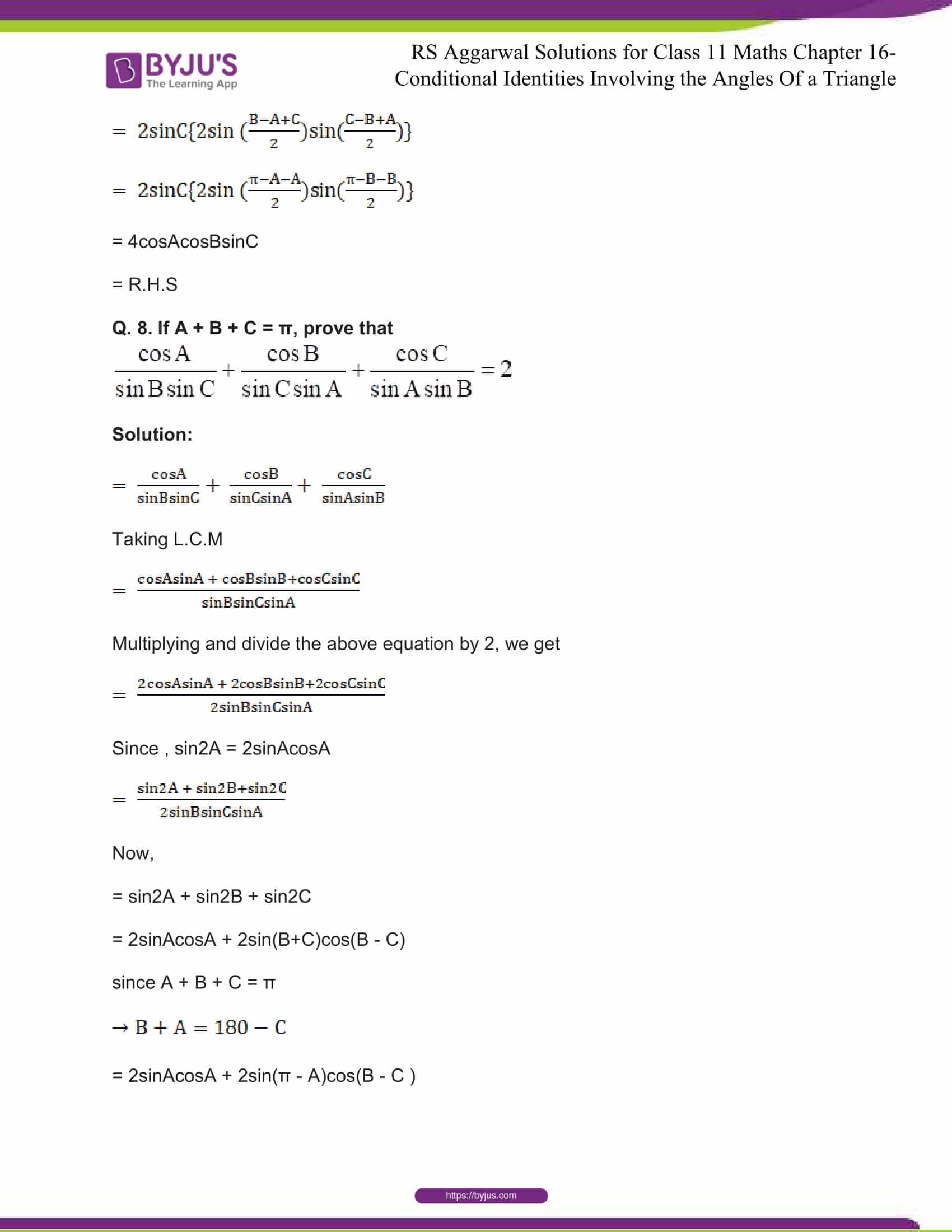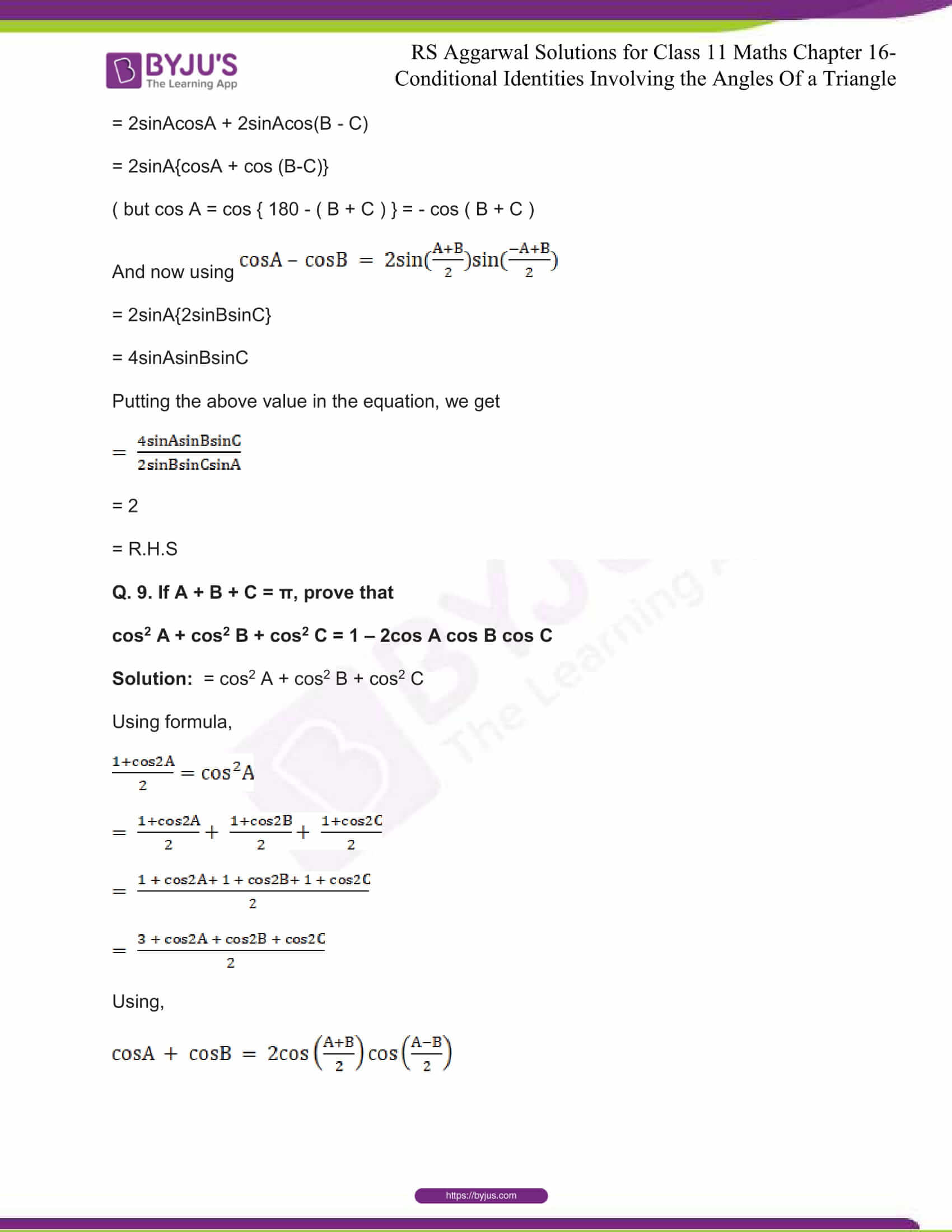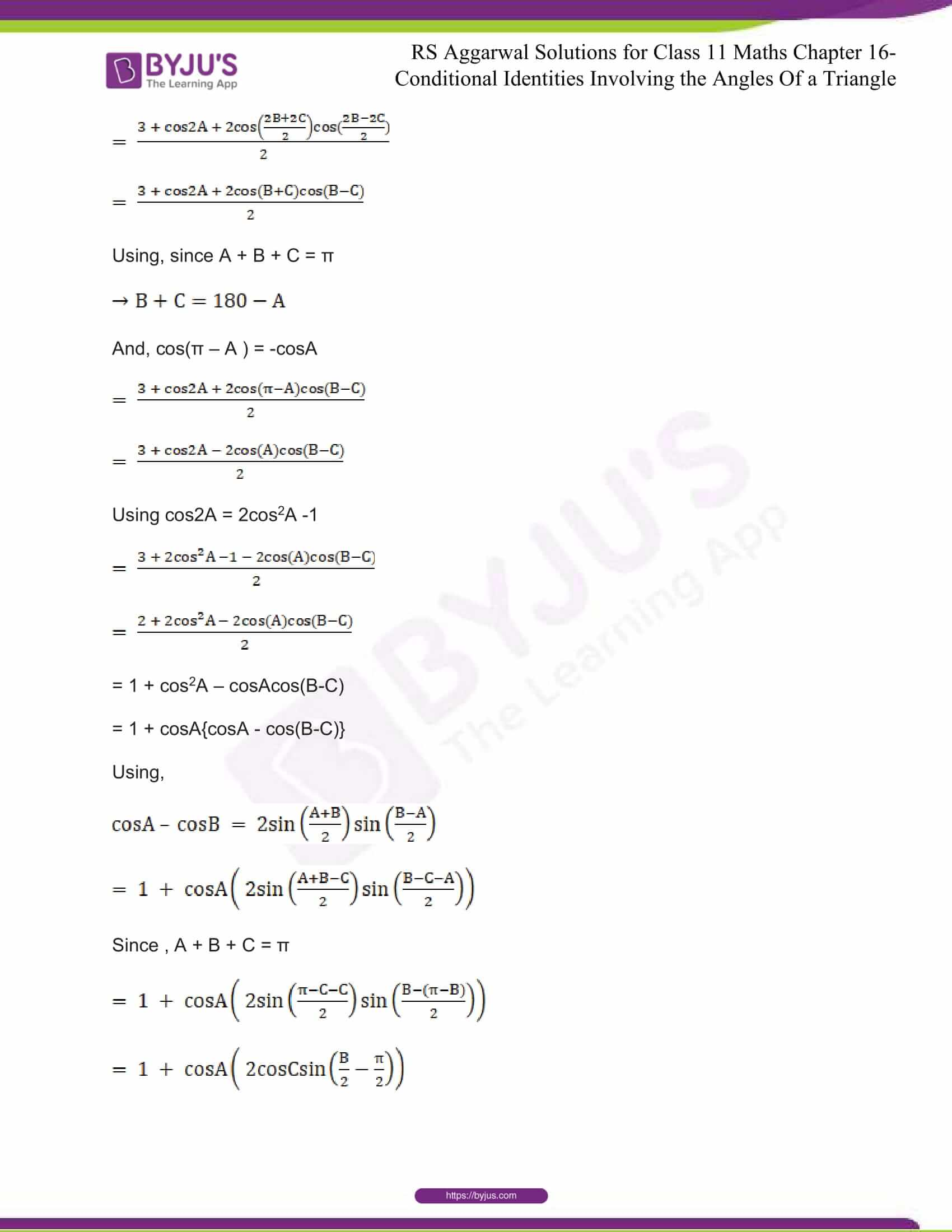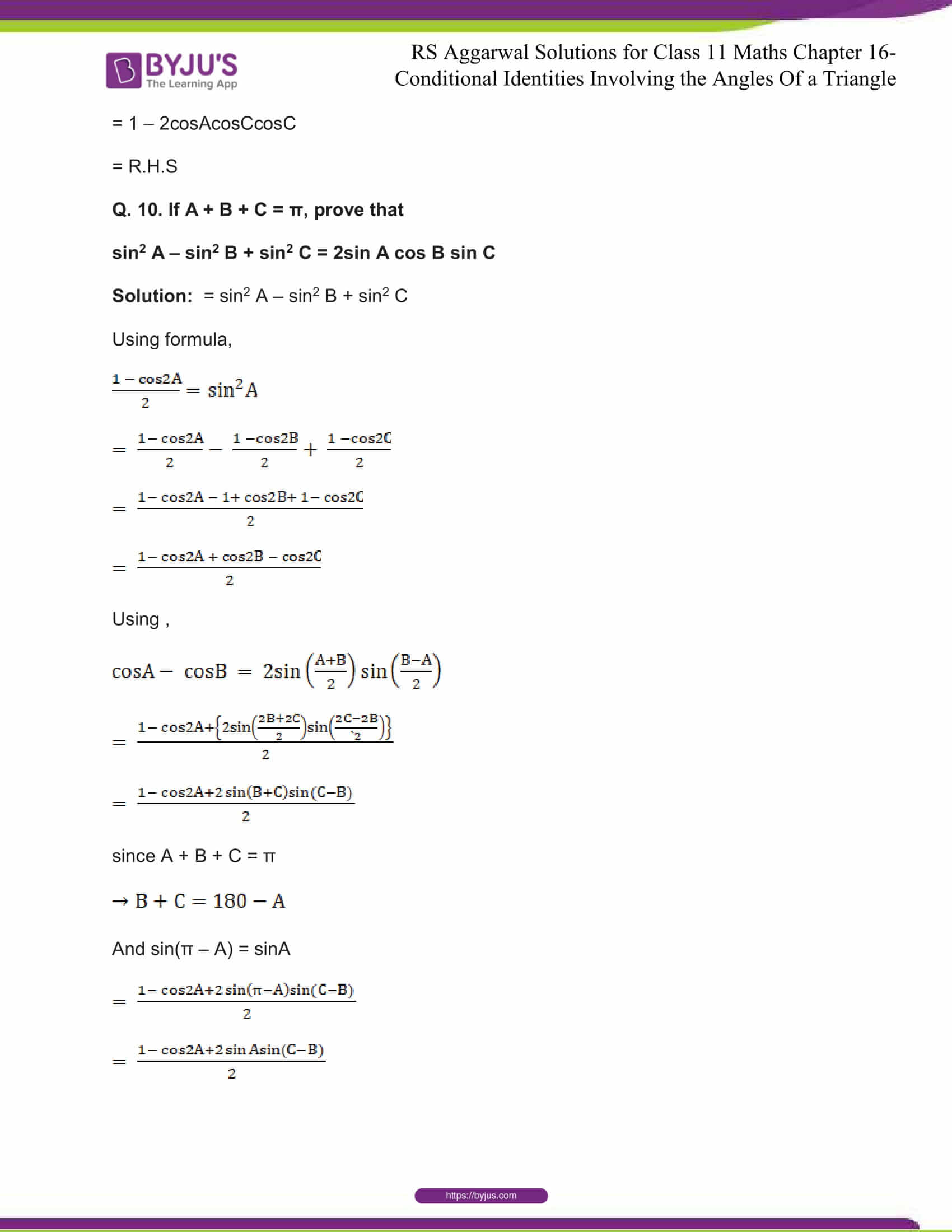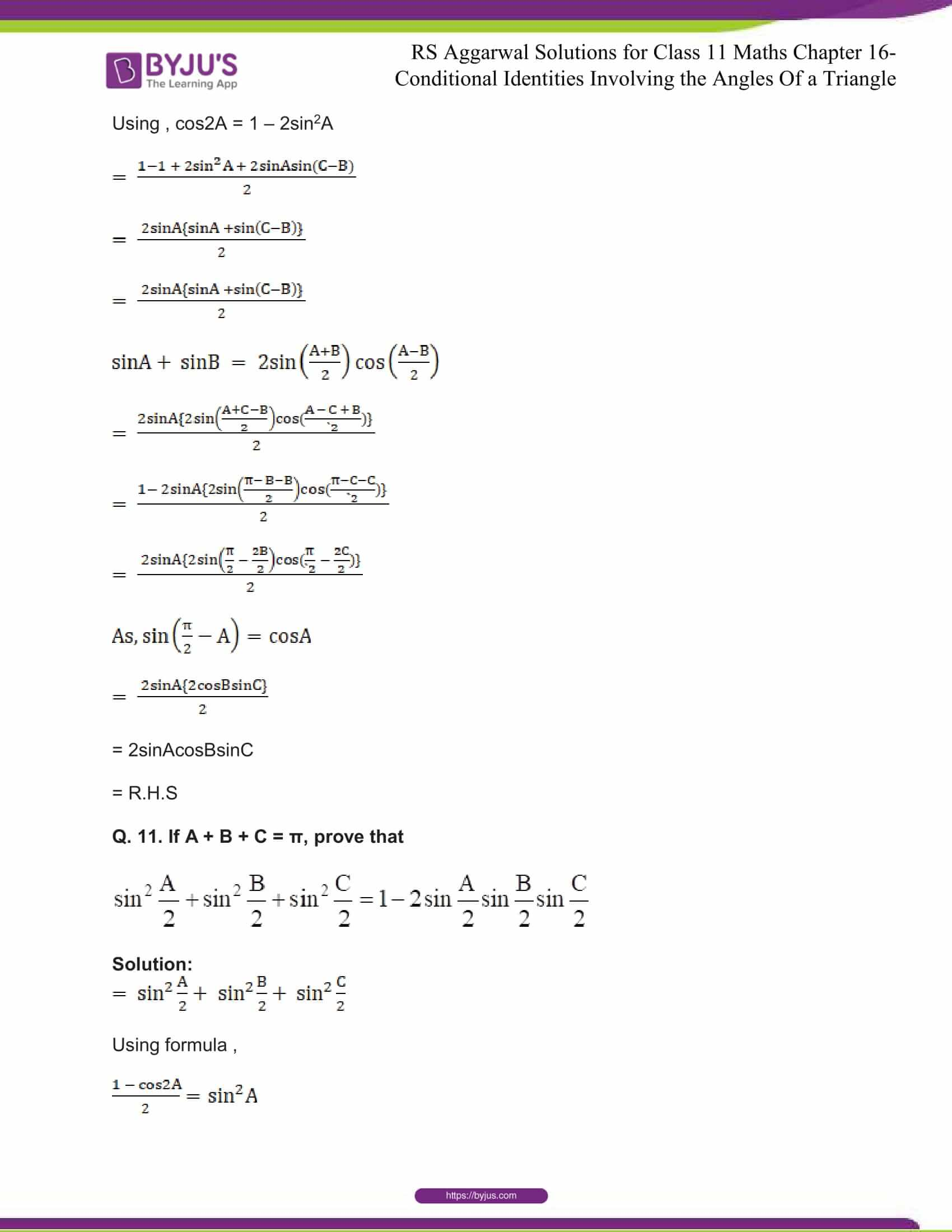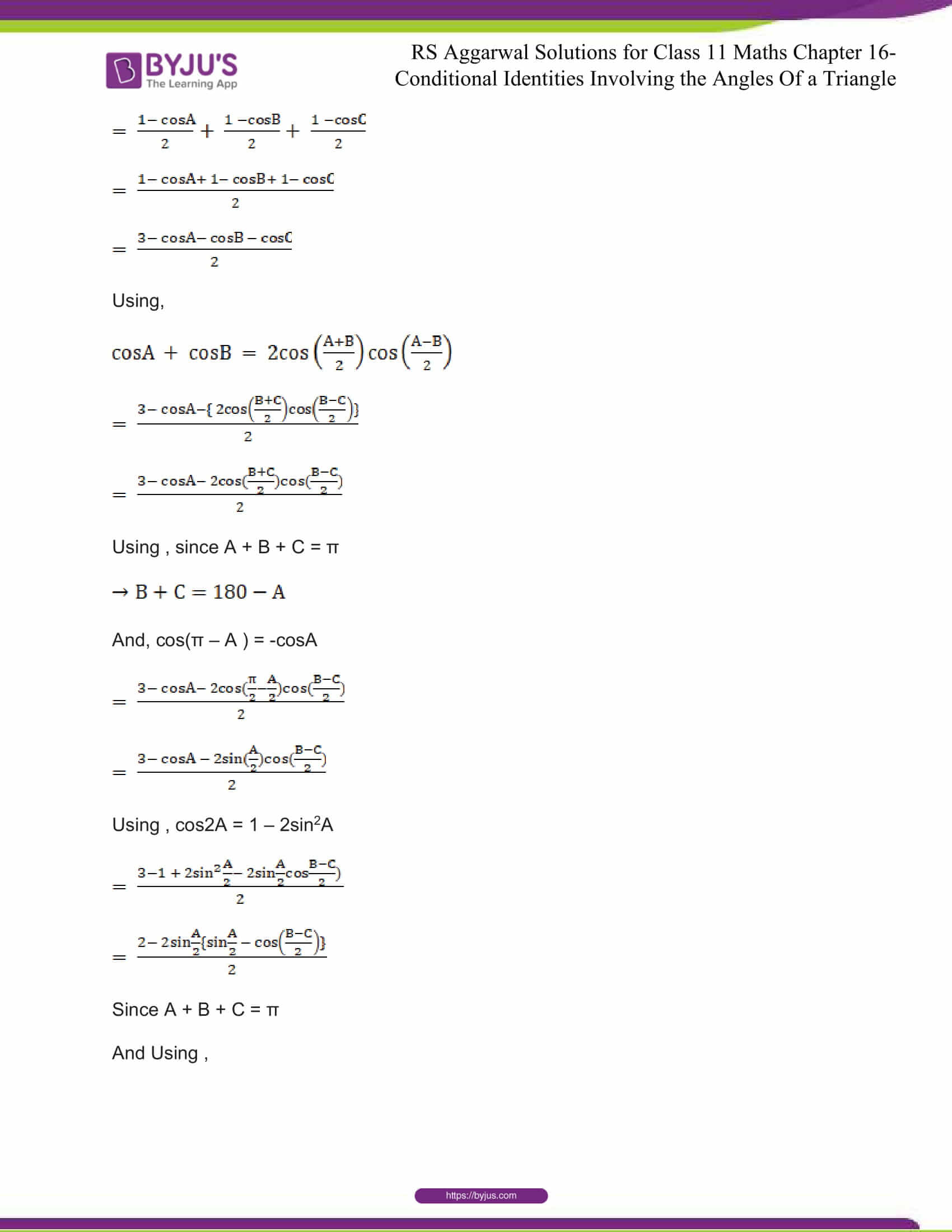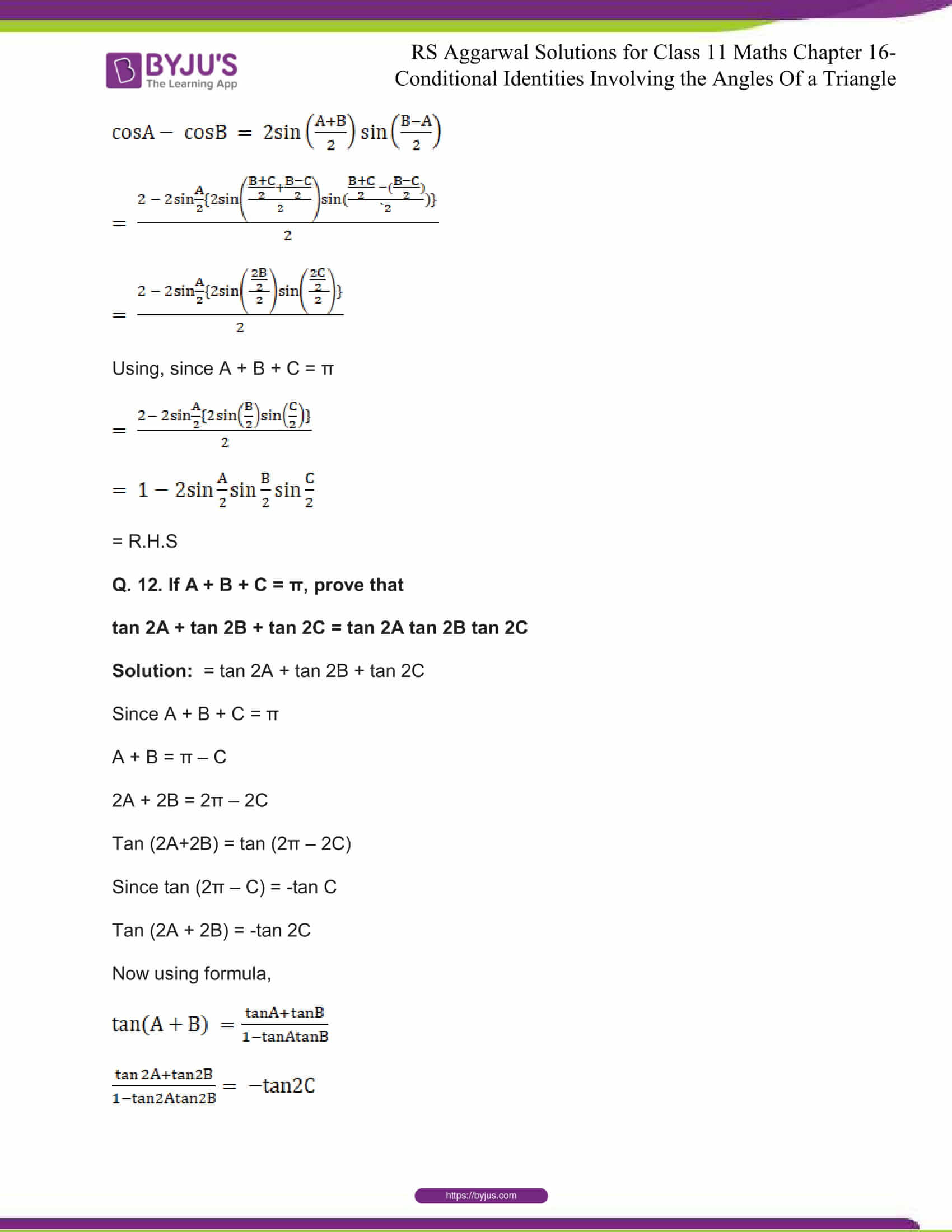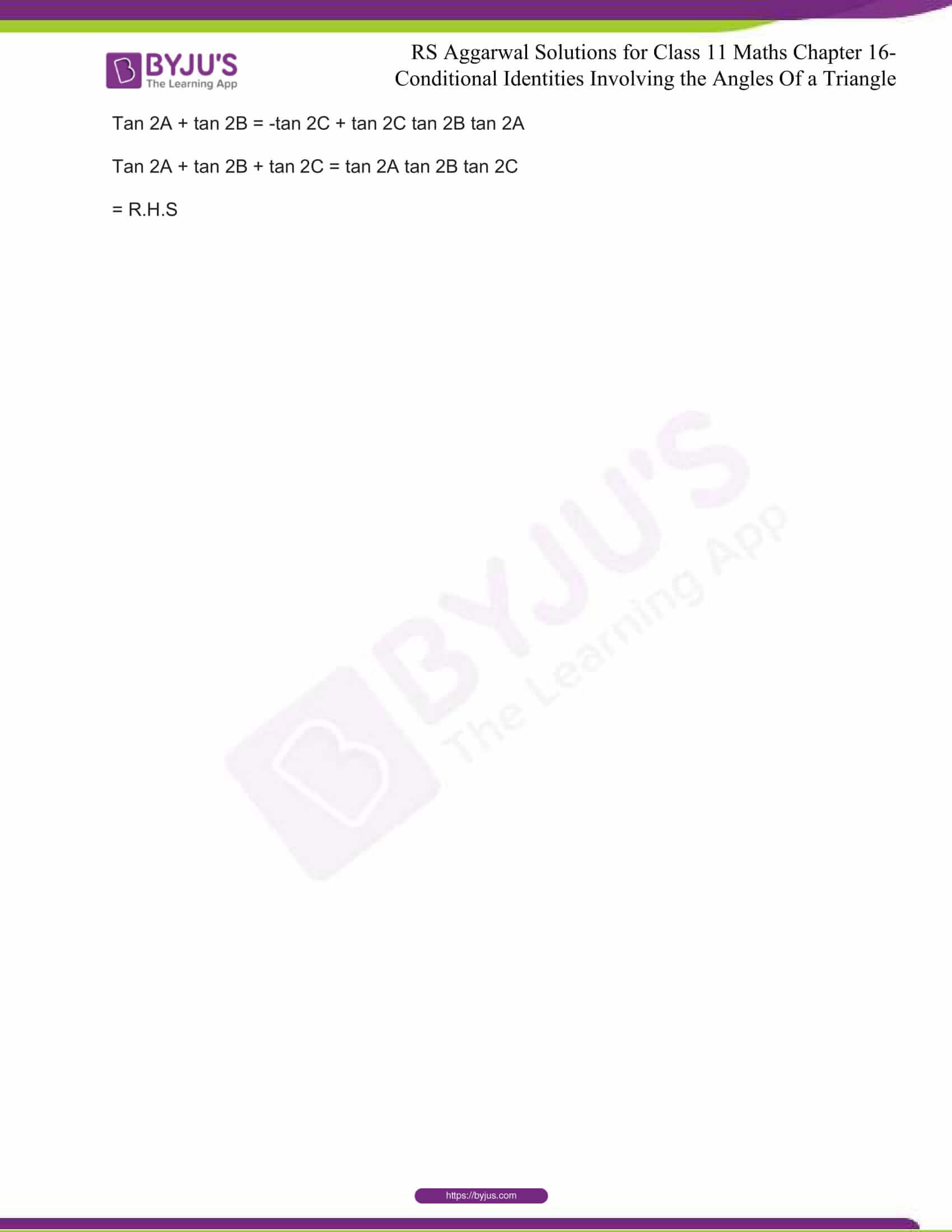## R S Aggarwal Solutions for Class 11 Maths Chapter 16- Conditional Identities Involving the Angles of a Triangle

Chapter 16, Conditional Identities Involving the Angles of a Triangle, consists of 1 exercise and the solutions given here contain answers to all the questions present in the exercise. Let us have a look at some of the topics that are being discussed in this chapter.

16.1 Identities involving sines and cosines

16.2 Identities involving squares of sines and cosines

16.3 Identities involving tangents

## R S Aggarwal Solutions for Class 11 Maths Chapter 16- Conditional Identities Involving the Angles of a Triangle

In conditional trigonometric identities, the specific relationships that exist among the angles involved are discussed. Some trigonometric identities are true for all the values of angles associated, satisfying the given conditions. These identities are called conditional trigonometric identities. Read and practice Chapter 16 of R S Aggarwal textbook to learn more about Conditional Identities Involving the Angles of a Triangle. Learn the R S Aggarwal Solutions for Class 11 effectively to attain excellent results in the examination.